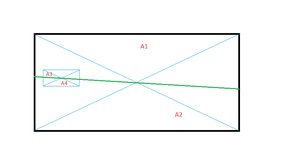Open In App

# Puzzle 48 | Rectangular Cardboard

Given, a rectangular cardboard with a rectangular piece removed(of any size and orientation) how one can cut the rectangular cardboard in two equal parts with one straight line cut. Solution: Using the fact: that “any line passing through the center of a rectangle divides the rectangle into two equal pieces.” One can cut the rectangular cardboard by the line joining the center of the original rectangular cardboard and the center of the removed piece, this will divide the rectangular cardboard into exactly two pieces. For example: refer to figureRectangle cut into equal halves by a single straight line

A1 = A2 ….(1)

and similarly A3=A4 …(2)

So, A1-A3 = A2-A4 [using both 1and 2]